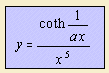## exponential

last updated: 2003-07-20The physicist Planck stated as a result of theoretical and experimental considerations a formula for the temperature radiation energy of a black body, as function of wavelength and temperature 1). This theory was one of the foundations of quantum physics. Crucial was Planck's assumption of packets of energy. Characteristic for the relationship is that for higher temperatures the wavelength with maximum radiation energy gets smaller. This fact could not be rightly understood with the classical theories.
In the curve the right side gives the radiation energy (y) as function of the wavelength (x). The parameter a is the temperature, apart from a constant (k / hc 2)).

The hyperbolic cotangent part in the formula is the Planck distribution.

notes

1) Kittel 1976 p. 128.

2) k: Boltzmann constant; h: Heisenberg constant; c: light velocity.